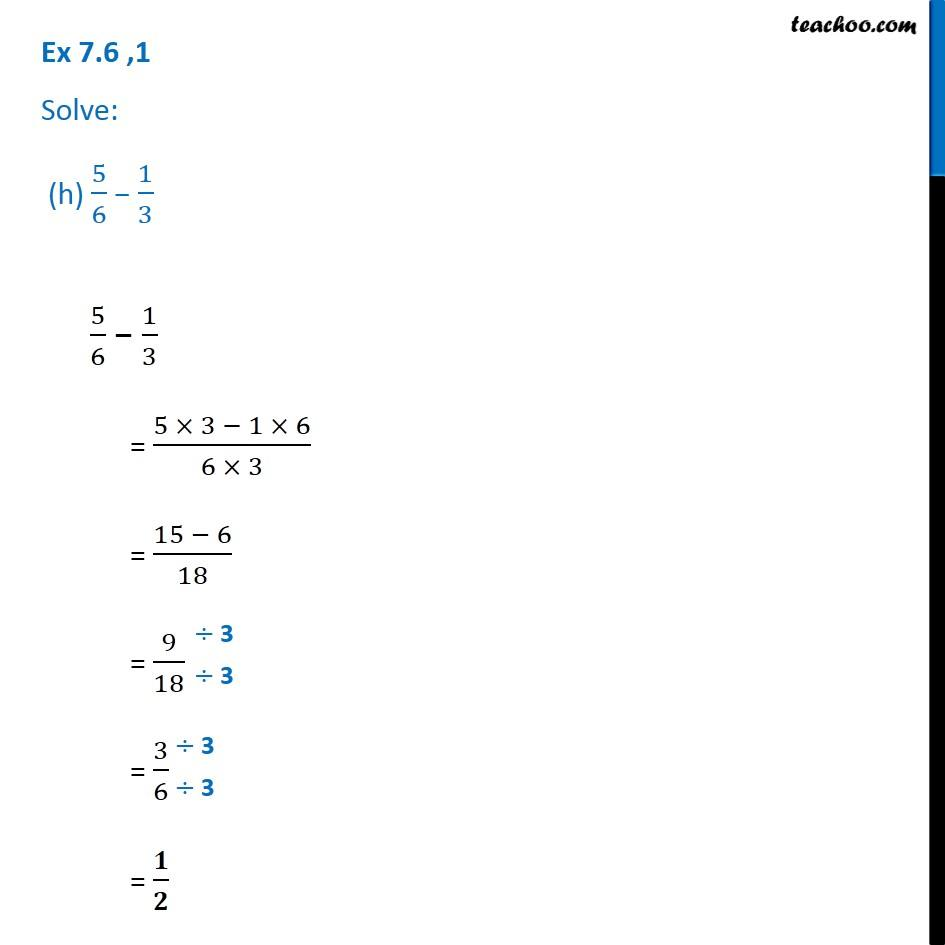1. Chapter 7 Class 6 Fractions
2. Serial order wise
3. Ex 7.6

Transcript

Ex 7.6 ,1 Solve: (h) 5/6 1/3 5/6 1/3 = (5 3 1 6)/(6 3) = (15 6)/18 = 9/18 = 3/6 = /